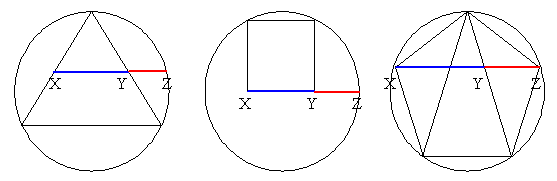#### You may also like### Gold Again

Without using a calculator, computer or tables find the exact values of cos36cos72 and also cos36 - cos72.### Pythagorean Golden Means

Show that the arithmetic mean, geometric mean and harmonic mean of a and b can be the lengths of the sides of a right-angles triangle if and only if a = bx^3, where x is the Golden Ratio.### Golden Triangle

Three triangles ABC, CBD and ABD (where D is a point on AC) are all isosceles. Find all the angles. Prove that the ratio of AB to BC is equal to the golden ratio.

# Gold Yet Again

##### Age 16 to 18Challenge Level

Nick Lord says that this problem is immediately enticing for students (and for him) and there are many possible approaches to explore and compare.In the equilateral triangle inscribed in the circle, $X$ and $Y$ are the midpoints of the edges. Find the ratio${XZ\over XY}$.

The square sits on a diameter of the circle. Find the ratio ${XZ\over XY}$.

The regular pentagon is inscribed in the circle. Find the ratio ${XZ\over XY}$.